Documentation

### This is machine translation

Mouseover text to see original. Click the button below to return to the English version of the page.

Note: This page has been translated by MathWorks. Click here to see
To view all translated materials including this page, select Country from the country navigator on the bottom of this page.

# rcosdesign

Raised cosine FIR pulse-shaping filter design

## Syntax

``b = rcosdesign(beta,span,sps)``
``b = rcosdesign(beta,span,sps,shape)``

## Description

example

````b = rcosdesign(beta,span,sps)` returns the coefficients, `b`, that correspond to a square-root raised cosine FIR filter with rolloff factor specified by `beta`. The filter is truncated to `span` symbols, and each symbol period contains `sps` samples. The order of the filter, `sps*span`, must be even. The filter energy is 1.```

example

````b = rcosdesign(beta,span,sps,shape)` returns a square-root raised cosine filter when you set `shape` to `'sqrt'` and a normal raised cosine FIR filter when you set `shape` to `'normal'`.```

## Examples

collapse all

Specify a rolloff factor of 0.25. Truncate the filter to 6 symbols and represent each symbol with 4 samples. Verify that `'sqrt'` is the default value of the `shape` parameter.

```h = rcosdesign(0.25,6,4); mx = max(abs(h-rcosdesign(0.25,6,4,'sqrt')))```
```mx = 0 ```
`fvtool(h,'Analysis','impulse')`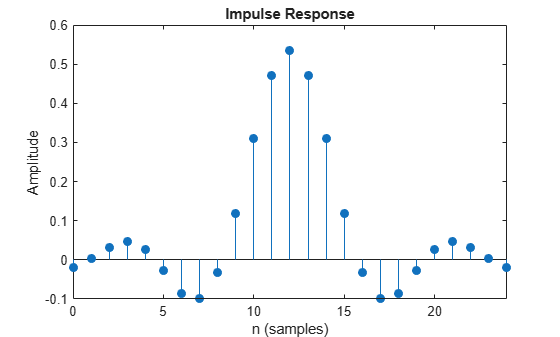Compare a normal raised cosine filter with a square-root cosine filter. An ideal (infinite-length) normal raised cosine pulse-shaping filter is equivalent to two ideal square-root raised cosine filters in cascade. Thus, the impulse response of an FIR normal filter should resemble that of a square-root filter convolved with itself.

Create a normal raised cosine filter with rolloff 0.25. Specify that this filter span 4 symbols with 3 samples per symbol.

```rf = 0.25; span = 4; sps = 3; h1 = rcosdesign(rf,span,sps,'normal'); fvtool(h1,'impulse')```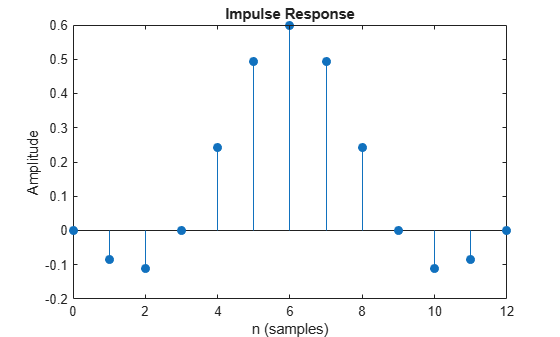The normal filter has zero crossings at integer multiples of `sps`. It thus satisfies Nyquist's criterion for zero intersymbol interference. The square-root filter, however, does not:

```h2 = rcosdesign(rf,span,sps,'sqrt'); fvtool(h2,'impulse')```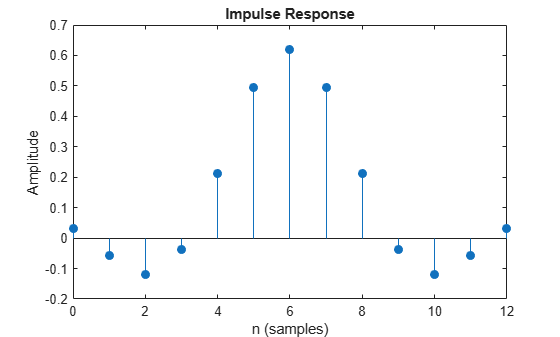Convolve the square-root filter with itself. Truncate the impulse response outward from the maximum so it has the same length as `h1`. Normalize the response using the maximum. Then, compare the convolved square-root filter to the normal filter.

```h3 = conv(h2,h2); p2 = ceil(length(h3)/2); m2 = ceil(p2-length(h1)/2); M2 = floor(p2+length(h1)/2); ct = h3(m2:M2); stem([h1/max(abs(h1));ct/max(abs(ct))]','filled') xlabel('Samples') ylabel('Normalized amplitude') legend('h1','h2 * h2')```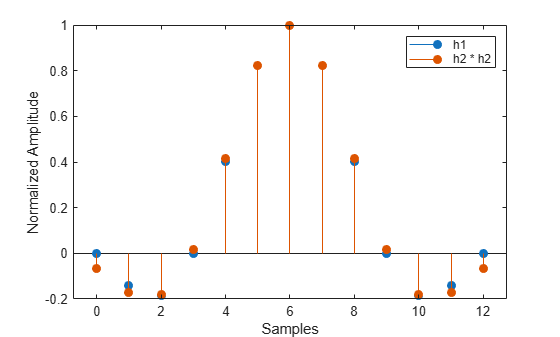The convolved response does not coincide with the normal filter because of its finite length. Increase `span` to obtain closer agreement between the responses and better compliance with the Nyquist criterion.

This example shows how to pass a signal through a square-root, raised cosine filter.

Specify the filter parameters.

```rolloff = 0.25; % Rolloff factor span = 6; % Filter span in symbols sps = 4; % Samples per symbol```

Generate the square-root, raised cosine filter coefficients.

`b = rcosdesign(rolloff, span, sps);`

Create a vector of bipolar data.

`d = 2*randi([0 1], 100, 1) - 1;`

Upsample and filter the data for pulse shaping.

`x = upfirdn(d, b, sps);`

Add noise.

`r = x + randn(size(x))*0.01;`

Filter and downsample the received signal for matched filtering.

`y = upfirdn(r, b, 1, sps);`

This example shows how to interpolate and decimate signals using square-root, raised cosine filters designed with the `rcosdesign` function. This example requires the Communications Toolbox™ software.

Define the square-root raised cosine filter parameters. Define the signal constellation parameters.

```rolloff = 0.25; % Filter rolloff span = 6; % Filter span sps = 4; % Samples per symbol M = 4; % Size of the signal constellation k = log2(M); % Number of bits per symbol```

Generate the coefficients of the square-root raised cosine filter using the `rcosdesign` function.

`rrcFilter = rcosdesign(rolloff, span, sps);`

Generate 10000 data symbols using the `randi` function.

`data = randi([0 M-1], 10000, 1);`

Apply PSK modulation to the data symbols. Because the constellation size is 4, the modulation type is QPSK.

`modData = pskmod(data, M, pi/4);`

Using the `upfirdn` function, upsample and filter the input data.

`txSig = upfirdn(modData, rrcFilter, sps);`

Convert the Eb/N0 to SNR and then pass the signal through an AWGN channel.

```EbNo = 7; snr = EbNo + 10*log10(k) - 10*log10(sps); rxSig = awgn(txSig, snr, 'measured');```

Filter and downsample the received signal. Remove a portion of the signal to account for the filter delay.

```rxFilt = upfirdn(rxSig, rrcFilter, 1, sps); rxFilt = rxFilt(span+1:end-span);```

Create a scatterplot of the modulated data using the first 5000 symbols.

```hScatter = scatterplot(sqrt(sps)* ... rxSig(1:sps*5000),... sps,0,'g.'); hold on scatterplot(rxFilt(1:5000),1,0,'kx',hScatter) title('Received Signal, Before and After Filtering') legend('Before Filtering','After Filtering') axis([-3 3 -3 3]) % Set axis ranges hold off```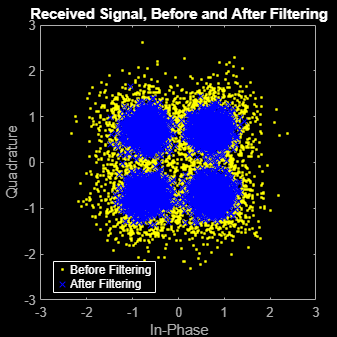## Input Arguments

collapse all

Rolloff factor, specified as a real nonnegative scalar not greater than 1. The rolloff factor determines the excess bandwidth of the filter. Zero rolloff corresponds to a brick-wall filter and unit rolloff to a pure raised cosine.

Data Types: `double` | `single`

Number of symbols, specified as a positive integer scalar.

Data Types: `double` | `single`

Number of samples per symbol (oversampling factor), specified as a positive integer scalar.

Data Types: `double` | `single`

Shape of the raised cosine window, specified as either `'normal'` or `'sqrt'`.

## Output Arguments

collapse all

Raised cosine filter coefficients, returned as a row vector.

Data Types: `double` | `single`

## Tips

• If you have a license for Communications Toolbox™ software, you can perform multirate raised cosine filtering with streaming behavior. To do so, use the System object™ filters, `comm.RaisedCosineTransmitFilter` and `comm.RaisedCosineReceiveFilter`.

 Tranter, William H., K. Sam Shanmugan, Theodore S. Rappaport, and Kurt L. Kosbar. Principles of Communication Systems Simulation with Wireless Applications. Upper Saddle River, NJ: Prentice Hall, 2004.